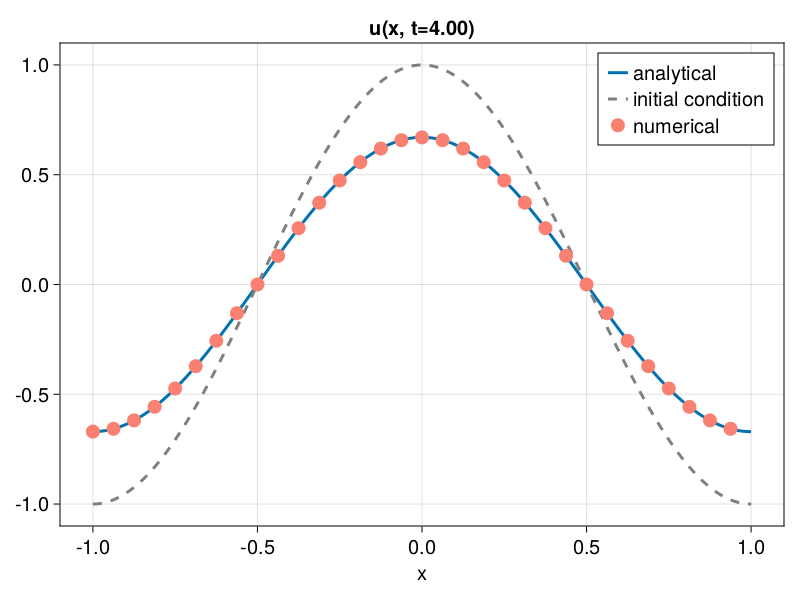# Problem

Everything needed to solve a PDE in FourierFlows.jl is gathered in a composite type named Problem. Problem contains various other composite types (see Problem for details).

Here, we demonstrate how we can construct a Problem to solve the simple 1D equation:

$$$\partial_t u(x, t) = - \alpha \, u(x, t) ,$$$

on domain $x \in [-1, 1]$.

First, we construct our grid

using FourierFlows

nx, Lx = 32, 2.0

grid = OneDGrid(nx, Lx)
OneDimensionalGrid
├─────────── Device: CPU
├──────── FloatType: Float64
├────────── size Lx: 2.0
├──── resolution nx: 32
├── grid spacing dx: 0.0625
├─────────── domain: x ∈ [-1.0, 0.9375]
└─ aliased fraction: 0.3333333333333333

Our problem has a parameter $\alpha$. Thus, we create a Params as:

struct Params <: AbstractParams
α :: Float64
end

and then we use the Params's constructor to populate our params with the parameter value, e.g., $\alpha = 0.1$:

α = 0.1

params = Params(α)
Parameters
└───── parameter: α -> Float64


The particular equation is so simple that it makes no difference performance-wise whether we time-step it in physical or in wavenumber space. For PDEs with nonlinear terms, time-stepping in wavenumber space is much more efficient. Thus, for demonstration purposes, we will time-step the equation in wavenumber space, i.e.,

$$$\partial_t \hat{u}(k, t) = - \alpha \, \hat{u}(k, t) .$$$

The variables involved are $u$ and its Fourier transform $\hat{u}$. Thus, we construct the vars as:

struct Vars <: AbstractVars
u :: Array{Float64, 1}
uh :: Array{Complex{Float64}, 1}
end

and, like before, we use the Vars's constructor to populate the vars with zero arrays,

vars = Vars(zeros(Float64, (grid.nx,)), zeros(Complex{Float64}, (grid.nkr,)))
Variables
├───── variable: u -> 32-element Vector{Float64}
└───── variable: uh -> 17-element Vector{ComplexF64}


Note that the Fourier transform of a real-valued array u is complex-valued. Also because we use the real Fourier transform, the array uh is smaller.

In this simple example our state variable is simply uh, i.e., sol = uh.

Next we need to construct the equation. Equation contains the linear coefficients for the linear part of the PDE, stored in an array L, and the function calcN!() that calculates the nonlinear terms from the state variable sol. In our case, our equation is linear and, therefore,

L = - params.α * ones(grid.nkr)

and

function calcN!(N, sol, t, clock, vars, params, grid)
@. N = 0

return nothing
end

Note that calcN!() needs to have the above argument structure. With L and calcN! in hand we can construct our problem's equation:

equation = FourierFlows.Equation(L, calcN!, grid)
Equation
├──────── linear coefficients: L
│                              ├───type: Float64
│                              └───size: (17,)
├───────────── nonlinear term: calcN!()
└─── type of state vector sol: ComplexF64

Last, we have to pick a time-stepper and a time-step dt and gather everything a FourierFlows's Problem:

stepper, dt = "ForwardEuler", 0.02

prob = FourierFlows.Problem(equation, stepper, dt, grid, vars, params)
Problem
├─────────── grid: grid (on CPU)
├───── parameters: params
├────── variables: vars
├─── state vector: sol
├─────── equation: eqn
├────────── clock: clock
└──── timestepper: ForwardEulerTimeStepper

Currently, the implemented time-steppers are ForwardEuler, AB3 (Adams-Basmforth 3rd order), RK4 (Runge-Kutta 4th order), and ETDRK4 (Exponential Time Differencing Runge-Kutta 4th order). Also, there exist the Filtered versions of all the above, in which a high-wavenumber filter is applied after every time-step.

By default, the Problem constructor takes sol a complex valued array filled with zeros with same size as L.

The prob.clock contains the time-step dt and the current step and time t of the simulation:

prob.clock
Clock
├─── timestep dt: 0.02
├────────── step: 0
└──────── time t: 0.0

Let's initiate our problem with, e.g., $u(x, 0) = \cos(\pi x)$, integrate up to $t = 4$ and compare our numerical solution with the analytic solution $u(x, t) = e^{-\alpha t} \cos(\pi x)$.

u0 = @. cos(π * grid.x)

using LinearAlgebra: mul!

mul!(prob.sol, grid.rfftplan, u0)

Since our time-step is chosen dt = 0.02, we need to step forward prob for $200$ time-steps to reach $t = 4$.

stepforward!(prob, 200)

Now let's transform our state vector sol back in physical space

using LinearAlgebra: ldiv!

ldiv!(prob.vars.u, grid.rfftplan, prob.sol)

and finally, let's plot our solution and compare with the analytic solution:

using CairoMakie, Printf

fig = Figure()
ax = Axis(fig[1, 1], xlabel = "x", title = @sprintf("u(x, t=%1.2f)", prob.clock.t))

x = range(-Lx/2, Lx/2, length=200)
lines!(ax, x, @. cos(π * x) * exp(-prob.params.α * 4); label = "analytical")
lines!(ax, x, @. cos(π * x); linestyle = :dash, color = :gray, label = "initial condition")

scatter!(ax, grid.x, prob.vars.u; markersize = 14, color = :salmon, label = "numerical")

axislegend()A good practice is to encompass all functions and type definitions related with a PDE under a single module, e.g.,

module MyPDE

...

end # end module

For a more elaborate example we urge you to have a look at the Diffusion module located at src/diffusion.jl and also the modules included in the child package GeophysicalFlows.jl.